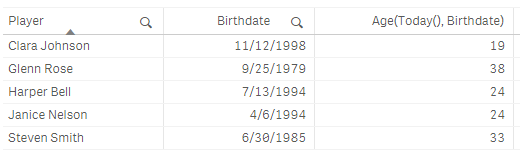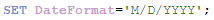Qlik Design Blog

All about product and Qlik solutions: scripting, data modeling, visual design, extensions, best practices, etc.

Announcements
QLIKWORLD LIVE! MAY 16 - 19TH, EARLY BIRD DISCOUNTS! REGISTER TODAYEmployee

Age Function

In this blog I thought I would share the Age function – a function that is not new to Qlik Sense or QlikView but new to me.  The Age function, which can be used in the script or in a chart expression, returns the age or the number of “completed years” based on a given date (timestamp in the syntax below) and a birthdate (date_of_birth in the syntax below).  The syntax for the Age function is as follows:

age(timestamp, date_of_birth)

Based on the timestamp value, the Age function will return the number of full years that have passed since the date_of_birth.  Let’s look at a few examples.  In the table below, there are 5 players along with their birthdate.  In the last column, I am calculating their age using the Age function.  I am using the Today() function for the first parameter – this will return the current date (7/13/2018) from the system clock.  The second parameter is the birthdate (a field in the data model) for the player.Notice that the date format for the current date and Birthdate are the same.  The format of dates in an app are based on the Set DateFormat statement at the beginning of the load script unless you opt to format them differently.  In this example, the DateFormat is set as follows:If I try to calculate the age based on the expression below, it will not work because the first parameter is not formatted properly based on the DateFormat (M/D/YYYY) I am using in the app.  In this case, the Age function will return null.Age can also be calculated based on a date other than the current date.  For instance, I can calculate age based on an event or the last day of the year.  The Age function is a very simple function to use and can be used in various scenarios other than age to calculate the number of years that have passed since a given date.

Thanks,

Jennell

1 Comment